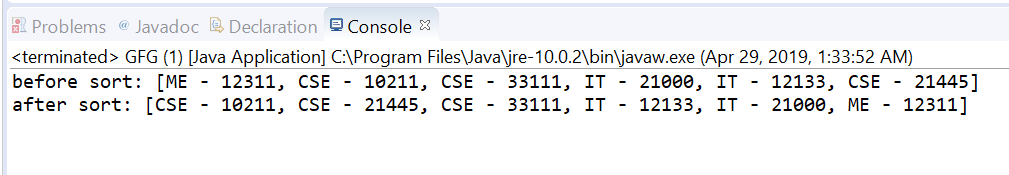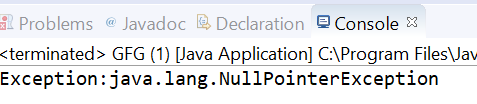# Comparator thenComparingLong() method in Java with examples

• Last Updated : 29 Apr, 2019

The thenComparingLong(java.util.function.ToLongFunction) method of Comparator Interface in Java returns a lexicographic-order comparator with a function that extracts a Long sort key.

Syntax:

```default Comparator <T> thenComparingLong(
ToLongFunction <T> keyExtractor)
```

Parameters: This method accepts keyExtractor which is the function used to extract the Long sort key.

Return value: This method returns a lexicographic-order comparator composed of this and then the Long sort key.

Exception: This method throws NullPointerException if the argument is null.

Below programs illustrate thenComparingLong(java.util.function.ToLongFunction) method:
Program 1:

 `// Java program to demonstrate Comparator``// thenComparingLong(ToLongFunction) method`` ` `import` `java.util.Arrays;``import` `java.util.Collections;``import` `java.util.Comparator;``import` `java.util.List;`` ` `public` `class` `GFG {``    ``public` `static` `void` `main(String... args)``    ``{``        ``List list = createUsers();``        ``System.out.printf(``"before sort: %s%n"``, list);``        ``Collections.sort(list,``                         ``Comparator``                             ``.comparing(Users::getDepartment)``                             ``.thenComparingLong(Users::getId));``        ``System.out.printf(``"after sort: %s%n"``, list);``    ``}`` ` `    ``private` `static` `List createUsers()``    ``{``        ``return` `Arrays.asList(``            ``new` `Users(``12311``, ``"ME"``),``            ``new` `Users(``10211``, ``"CSE"``),``            ``new` `Users(``33111``, ``"CSE"``),``            ``new` `Users(``21000``, ``"IT"``),``            ``new` `Users(``12133``, ``"IT"``),``            ``new` `Users(``21445``, ``"CSE"``));``    ``}`` ` `    ``private` `static` `class` `Users {``        ``private` `long` `id;``        ``private` `String department;`` ` `        ``public` `Users(``long` `id, String department)``        ``{``            ``this``.id = id;``            ``this``.department = department;``        ``}`` ` `        ``public` `long` `getId()``        ``{``            ``return` `id;``        ``}`` ` `        ``public` `void` `setId(``long` `id)``        ``{``            ``this``.id = id;``        ``}`` ` `        ``public` `String getDepartment()``        ``{``            ``return` `department;``        ``}`` ` `        ``public` `void` `setDepartment(String department)``        ``{``            ``this``.department = department;``        ``}`` ` `        ``@Override``        ``public` `String toString()``        ``{``            ``return` `department + ``" - "` `+ id;``        ``}``    ``}``}`

The output printed on console of IDE is shown below.
Output:You can see in example first sorting is done on department wise and if the department is same then ID wise.

Program 2:

 `// Java program to demonstrate Comparator``// thenComparingLong(ToLongFunction) method`` ` `import` `java.util.Arrays;``import` `java.util.Comparator;``import` `java.util.List;`` ` `public` `class` `GFG {``    ``public` `static` `void` `main(String... args)``    ``{`` ` `        ``List list``            ``= Arrays.asList(``"KKR"``, ``"CSK"``,``                            ``"MI"``, ``"KXIP"``, ``"RCB"``,``                            ``"SRH"``, ``"DC"``, ``"RR"``);`` ` `        ``try` `{`` ` `            ``// apply thenComparingLong``            ``Comparator.comparing(list::get)``                ``.thenComparingLong(``null``);``        ``}``        ``catch` `(Exception e) {`` ` `            ``System.out.printf(``"Exception:"` `+ e);``        ``}``    ``}``}`

The output printed on console is shown below.
Output:My Personal Notes arrow_drop_up